# Loan Analysis

A measure of loan safety that estimates the likelihood a borrower will repay the loan

Many parallels exist between loan formulae and PV models. Loan interest rates are like opportunity costs, loan amounts are like investments, and loan payments are like the cash flow from an investment.Because of these and other similarities between PV models and loan formulae, we may utilize PV tools to examine various types of loans and interest rates. The following article discusses different interest rate definitions.

This article will also explain the link between loan length and loan payment amount. Finally, we will determine loan refinancing break-even points and effective interest rates for various disguised interest rate loans.

Some loans need a more comprehensive examination than others. Long-term fixed-asset loans demand a more detailed investigation than short-term working capital loans.

Individual loan analysis and follow-up visits constitute the majority of the guarantee for the institution; thus, the study must be more detailed.

Group loans delegate most of this obligation to the clients and hence do not require in-depth investigation.

Loan analysis provides the creditor with a measure of loan safety by estimating the likelihood that the borrower will repay the loan (principal and interest).

Follow-up visits to potential borrowers, particularly for individual loans, assist the lending institution in gauging the success of the investments or assets expected to produce revenue to help pay the loans.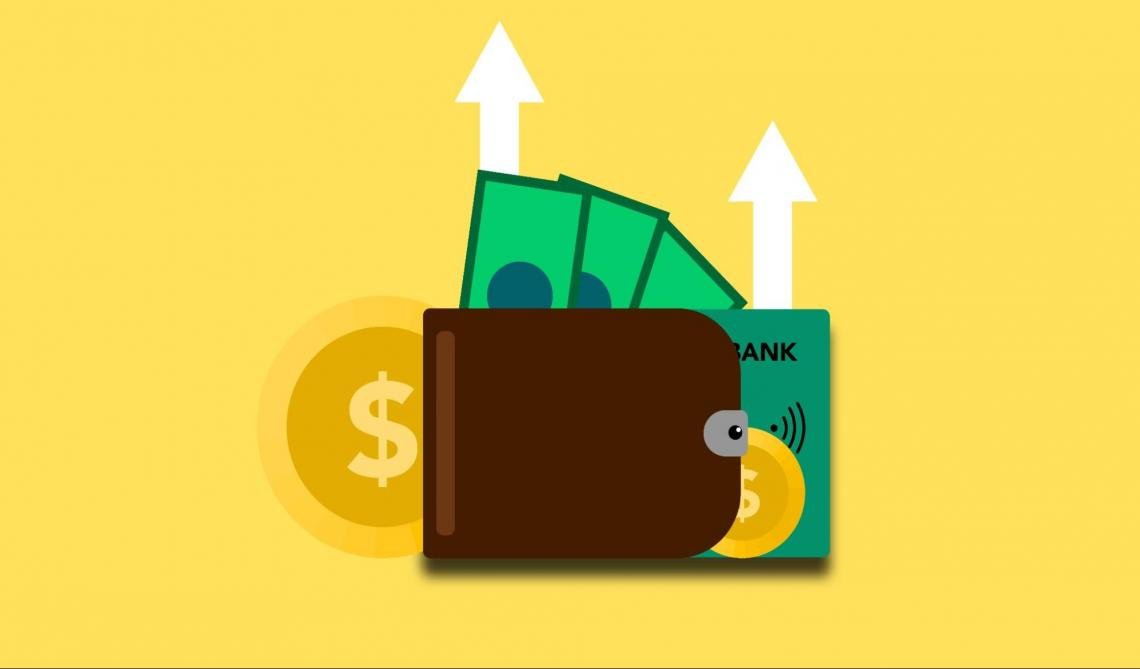Furthermore, a positive cash balance on the bank account statement suggests that the borrower has some money. It also demonstrates how successfully the applicant's financial flow is controlled.

It is simple to label a borrower as "responsible" if he keeps a positive cash balance for several months since this improves the likelihood that he will return the loan in the future. On the other hand, poor cash balances on the bank statement demonstrate the applicant's mishandling of funds and cast them in a negative light.

If you're thinking about the optimal amount of cash balance a borrower should keep in their bank accounts, you should know that it depends on the loan amount they have applied for.

As a general rule, the larger the loan application amount, the greater the required balance.

## Comparing the Actuarial Rate, Annual Percentage Rate (APR), and Effective Interest Rate

Loans have at least three types of interest rates that are closely connected. These rates, as well as their most regularly used synonyms, are mentioned below. The name of the interest rate used in this chapter is italicized. They are as follows:

(1) The actuarial rate, compound rate, real rate, or periodic rate (rf);

(2) The Annual Percentage Rate (APR), annual rate, or nominal rate (r/m).

(3) The effective interest rate or effective annual rate (re).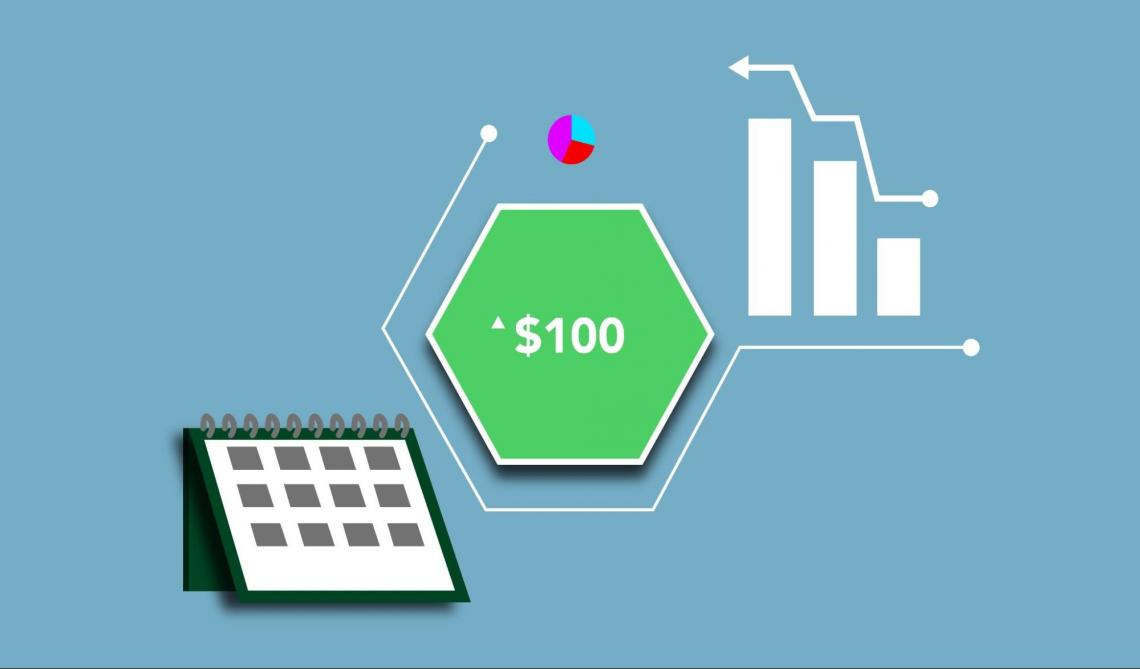The actuarial rate

Interest may be calculated and levied more than once a year in financial transactions. Savings account interest, for example, is normally computed daily, although many corporate bonds pay interest semiannually.

An actuarial interest rate is the interest rate used in calculations for periods of less than one year. The actuarial rate is the interest rate per compounding period or the interest rate per conversion period.

It is the actuarial rate at which interest is charged on the principal sum during each consecutive conversion period. Consider a 1% actuarial rate levied monthly on a \$1,000 investment.

In this scenario, the interest of 1% of \$1,000, or \$10, is levied in the first month of the loan. In the second month, interest on \$1,010 equals \$10.10, and so on. Let rf stand in for the Annual Percentage Rate (APR).

Let m represent the number of times interest is computed or charged during the year. As a result, m matches the number of compounding periods in a year. The actuarial rate, compound rate, genuine rate, or periodic rate is represented by the rf/m ratio.By expressing the actuarial rate annually, we may calculate the APR from the actuarial rates. To convert an actuarial rate to an APR, multiply the rate by m.

In the above example, we increase the actuarial rate of 1% per month by 12 to get an APR of 12%. The actuarial rate and the APR are equivalent when the compound or conversion period is one year long.

Define two savings banks with identical APRs. The sole distinction is that institution A provides monthly compounding of interest whereas institution B provides annual compounding. Which should the saver choose?

Monthly compounding is favored since the saver is interested in money generated throughout the year. With Institution B, interest is earned solely on principle saved and interest earned in prior years throughout the year.Effective interest rates

Effective interest rates, abbreviated as re, are the yearly interest rates charged.

When comparing APRs with differing numbers of compound periods each year, the actuarial rates should be translated to their effective interest rates. The effective rate is calculated by compounding the actuarial rate over one year.

The discrepancy between the APR and the effective rate grows as the number of compounding periods each year grows. It is simple to outline the connections between an actuarial rate, an APR, and an effective rate.

Let m be the number of compounding periods each year, rf the annual percentage rate, rf/m the actuarial rate, and re the effective rate. The following is the connection between the effective rate re, the APR rate rf, and the actuarial rate rf/m: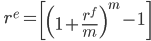(17.1)

When m = 1, the effective interest rate, APR rate, and actuarial rate are all identical; however, when m is not 1, the rates are no longer equal. Assume we want to determine the re assuming rf is compounded quarterly.

Let m = 4 and rf = 12 to solve this issue while using Substitution. Using Microsoft Excel and the values 12 for rf and 4 for m in equation (17.1), we obtain the results shown in Table 17.1. We input the function fx = ((1 + (B1 / B2))B2) – 1 in cell B3, which returns 12.55 percent.

Table 17.3: Calculating a continuously compounded effective interest rate.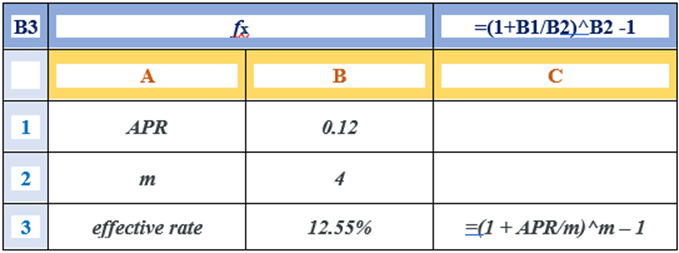The effective rate is found as previously when m is raised to 12, or monthly compounding periods. In the equation, let m = 12 and rf = 12. (17.1).

[(1 + 0.12/12)12 - 1]

To answer this problem in Excel, change cell B2 to 12 and calculate the effective interest rate, which equals 12.68 percent, as shown in Table 17.2.

Table 17.2: Calculating a monthly compounded interest rate.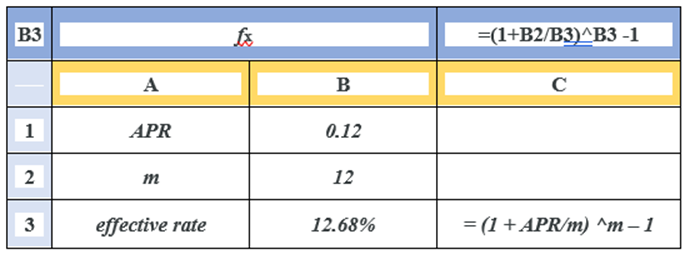Allowing a high number of periods to be compounded results in a particular compounding formula. This concept is stated as follows:

(17.3)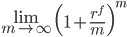That is, when m approaches infinity, the effective rate re equals:

(17.4)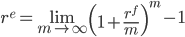Excel's EXP function is utilized to solve this problem. The function EXP(1) in Excel returns Euler's number "e," and 12 percent compounded continuously may be calculated as

fx = EXP(.12) – 1 = 12.75

Using the preceding cell identification, we would enter the following computation in B3 to obtain the value of 12 percent continuously compounding: EXP(B1) - 1 = 12.75 fx.

Table 17.3: Calculating a continuous effective interest rate.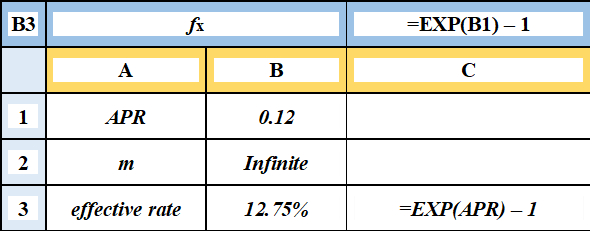## Loans with Constant Payments

We now utilize PV models to assess the most frequent loan, the continuous payment loan, once we have set interest rates in financial models.Constant payment loans are repaid over time with a succession of equal payments; refer to this as A. These payments might occur m times yearly for n years, for a total of mn payments.

Basic equality requires that the total loan installments discounted at the actuarial interest rate equal the loan amount.

The following is the relationship between the loan amount, L0, received in time zero, and payment A, made for mn periods at an actuarial interest rate (rf/m) commencing in period one:

L= A / (1+rf/m) +  A / (1+rf/m)+  ... + A / (1+rf/m)mn          (17.5)

The actuarial rate (rf/m) is the IRR for the PV model in this calculation, and it is unique since loan payments are constant.

Fortunately, the computations indicated in equation (17.5) are simple to carry out in Excel. In the following example, we compute L0.

Example 17.1:

A loan with a fixed payment covers the loan amount. Assume a borrower can make consistent monthly payments of \$150 for the next 48 months (four years).

The borrower wants to know how much of a loan may be repaid if the APR interest rate is 5% and the actuarial rate is.05/12 =.42%.

Using the Excel formula in Table 17.4 below, we can solve for the loan amount supported by the continuous loan payment of \$150 using equation (17.5):

Table 17.4: Calculating the Constant Payment Loan Amount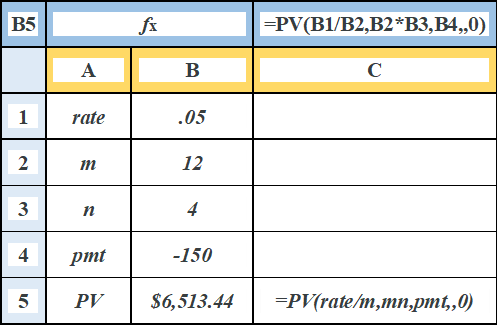The displayed answer is \$6,513.44. In other words, 48 monthly payments of \$150 on loan with a 5% APR interest rate and a monthly actuarial interest rate of .42 percent will repay a \$6,513.44 debt.

Fortunately, if the loan amount, length, and interest rate are known, Excel can also solve for the constant payment. Example 17.2 describes a loan with a continual payment.

Example 17.2:

• Loan Annuities with Constant Payments. Assume \$5,000 is borrowed from a lending institution for five years at a 12-percent APR or a 1% monthly actuarial rate.

• The loan will be paid back in 60 equal monthly payments. What is the payment or annuity required to pay off the loan?

• In Table 17.5, we solve the loan payment using Excel's PMT function.

• The displayed result is –\$111.22, which implies that 60 payments of \$111.22 on loan with a 12 percent APR interest rate and a monthly actuarial interest rate of 1% would repay a \$5,000 loan.

Table 17.5: Calculation of constant loan payments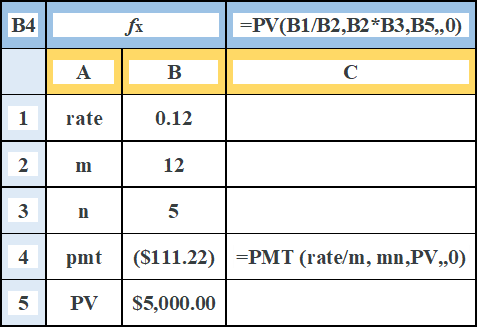Finally, consider a loan situation in which the unknown variable is the loan term. Example 17.3 introduces such an issue, and Table 17.6 contains the Excel solution.

Example 17.3:

• The loan duration is necessary to pay off a continuous payment loan. Assume a borrower can make consistent monthly payments of \$150.

• The borrower wants to know how many monthly payments will be necessary to pay off an \$8,000 loan if the APR interest rate is 6% and the monthly actuarial rate is.5%.

• The problem is solved using the NPER function in Excel, as shown in Table 17.6.

• The displayed result is 62.19, which implies that 62 regular payments and one partial payment will be required to retire an \$8,000 loan if the APR interest rate is 6%.

Table: 17.6: Calculating the Number of Payments for Retiring a Loan## Comparing Interest Paid, Loan Term, and Payment Amounts for Constant Payment Loans

The loan period and total interest payments have a significant relationship. For example, consider a \$30,000 loan with a 15% APR that will be repaid in monthly installments over 30 years. This loan has a monthly payment of \$379.33.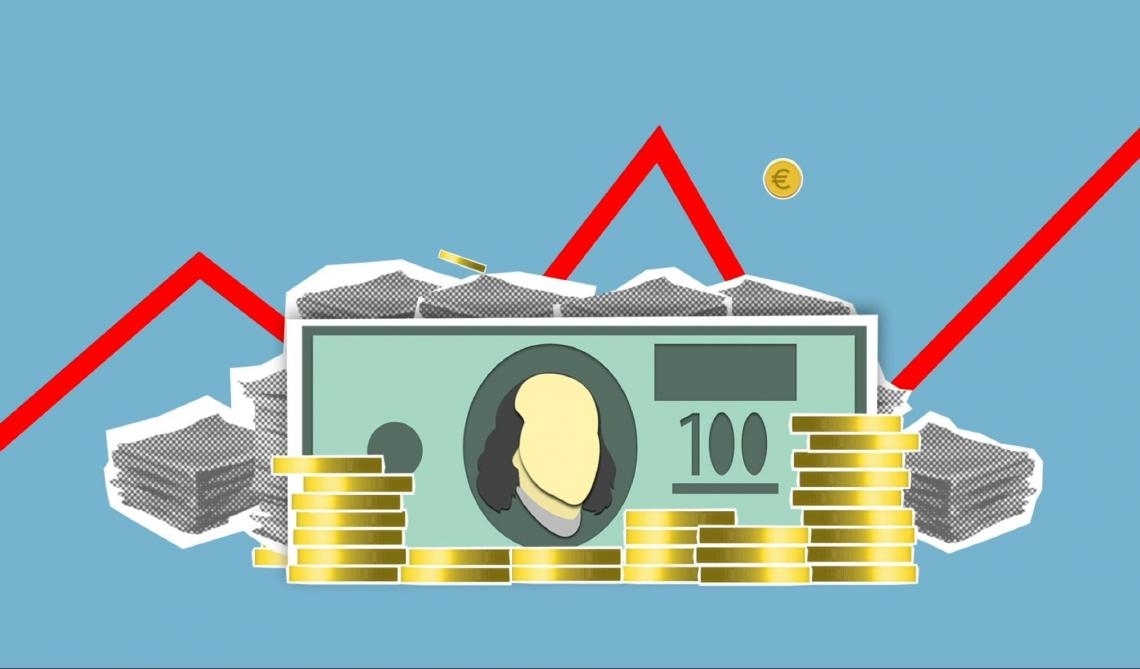The total interest (TI) paid on a constant payment loan is calculated by multiplying the constant loan payment by the loan term. A multiplied by the loan duration mn, minus the loan amount L0:

TI = (A)(mn) - L= (\$379.33)(360) - \$30,000 = \$106,560      (17.6)

The total interest paid in this example is \$106,560.

Increasing the monthly amount by 10% to \$417.27 decreases the loan period by 48% to slightly over 15.36 years (verify the results above using Excel). Meanwhile, the total interest paid has been lowered by 56% to \$46,961.

The term decrease due to a higher loan payment is not always as significant. For example, if the aforementioned loan had an APR of 8%, the monthly payment would be \$220.13 rather than \$379.22.

By increasing the payment by 10%, the loan duration is reduced by just 27%, from 30 years to 21.93 years, while the total interest paid is reduced by 32%, from \$49,247 to \$33,722.

It would be interesting to understand how altering the loan duration impacts payment size and total interest paid.

As mn increases, payment A approaches the interest cost each period. The smallest payment conceivable equals the interest imposed on the outstanding loan sum.

If the borrower wishes to pay only interest, the suitable term allows the borrower to return only interest. In contrast, the smallest payback period is one.

There is a trade-off between the loan payment size and the loan term.

The point elasticity of term, measured in years n concerning payment A, is the percentage change in term n in reaction to a 1% change in the loan payment. The elasticity term, E(n, A), has been computed as follows:

En.A = 1 - erm / rn < 0      (17.7)

A 30-year loan with a 15% APR, for example, would have term elasticity equal to

En.A = 1 - e(0.15)(30)/ (0.15)(30) = -19.78% < 0      (17.8)

In other words, raising the payment by 1% decreases the period by about 19.78%.

In contrast, the arc elasticity, rather than the point elasticity, compares the percentage change in the loan term to a 10% increase in the loan payment and calculates.

The percentage change in the term is 48% or an arc elasticity of term of 4.8 percent.

It is worth noting that a point elasticity of 19.78 percent versus an arc elasticity of 48 percent results from comparing huge changes in loan payments of 10% with little changes in loan payments (e.g., .00001 percent ).

Table 17.7 of point elasticities is shown below.

Table 17.7: Point elasticity measurements for various loan terms and interest rates.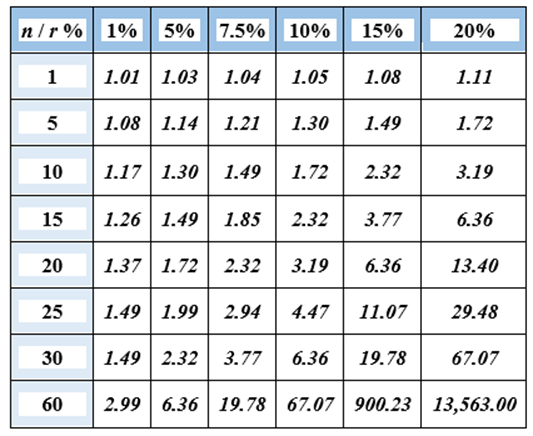Example 17.4:

• Loan Payment and Term Trade-Offs Lucy's Landlord is funding a property makeover. She requires a \$28,000 loan. Her lender gives her a 20-year loan at the current interest rate of 15%.

• Her yearly payout is calculated to be \$4,473.32. If she increases her payment by 1% to \$4,518.05, her sentence is shortened to 19 years, a 5% decrease.

• This percentage reduction is approximately equal to the tabulated value of 6.36 in Table 17.1, located at the intersection of row 20 and column 0.15.

• Large percentage changes in A, such as 10%, may not be adequately reflected in the point elasticities table.

• This is due to the significant percentage changes in n concerning A compared to the minor changes in n for A to create the table.

## Creating an Amortization Table for Constant Payment Loans

Originally, the term "amortize" meant "to kill." As a result, when we amortize a debt, we effectively kill or extinguish it by making regular payments-killing the loan, if you will.The amount of the payment devoted to paying off the loan-the principal component-and the amount of the payment devoted to paying the interest on the loan are continually changing, which is one element of the constant payment loan.

As the loan principal is decreased or eliminated, the amount of the payment devoted to interest charges decreases while the amount of the payment devoted to loan reduction increases.

When asked, lending institutions will give amortization tables outlining the amount of interest and principal paid on each payment throughout the life of the loan.

Fortunately, Excel gives us the necessary tools to generate our amortization tables.

Using Excel, calculate the principal component of the tth loan installment. The PPMT function in Excel may calculate the principal component Principale tth loan installment. The function is written as

PPMT(rate, per, nper, PV,,0)    (17.9)

Consider a \$25,000 loan that will be repaid in monthly installments over four years to demonstrate the PPMT function. The loan's annual percentage rate is 5%.

We'd want to know how much of the fifth payment (period) will be allocated to the principle of the loan. Table 17.8 displays the Excel solution.

Table 17.8: Calculating the Loan Principal on the tth Payment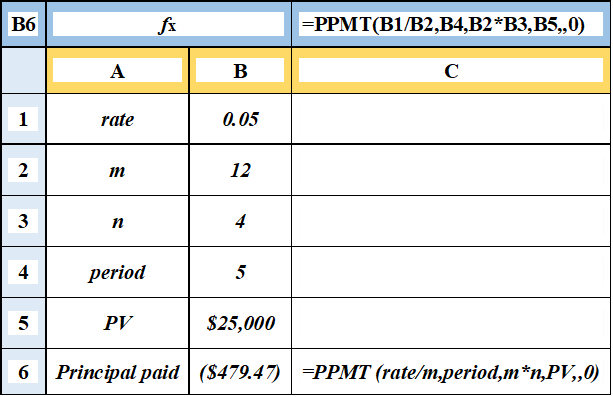The fifth payment of \$479.47 is applied to the outstanding loan principal. Furthermore, students may confirm that the major component of the 25th payment is \$521.05.

This is since the outstanding principal on which interest is levied reduces during the loan's duration. As interest rates fall, more of the loan payment may be allocated to the principal balance.

Using Excel, calculate the interest payment IP(t) on the tth loan installment. The IPMT function in Excel may be used to calculate the interest component of loan payment in the tth period, which is stated as:

IPMT (rate, per, nper, PV,,0)    (17.10)

To demonstrate the IPMT function, consider the following scenario: a loan of \$25,000, a period of 48 monthly installments at 5% APR, and a wish to calculate the interest paid on the fifth loan payment.

We show how to use Excel to find the interest paid on the tth period. In cell B8, the answer is recorded as \$54.68. Table 17.9 goes into much information about the solution.

Table 17.9: Calculating Loan Interest Paid on the tth Payment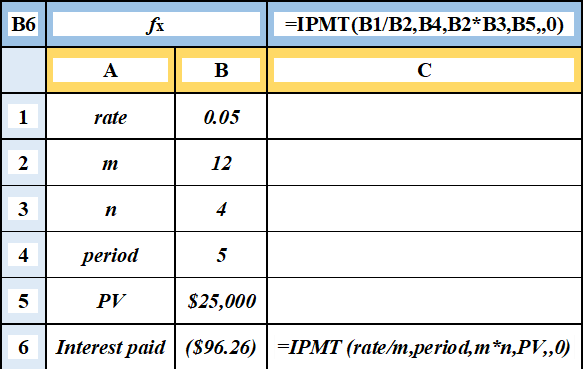The interest payment of \$96.26 plus the principal payment of \$479.47 equals the \$575.73 continuous loan payment.### Everything You Need To Master Excel Modeling

To Help You Thrive in the Most Prestigious Jobs on Wall Street.

Researched and authored by Fatemah Kamali | LinkedIn

Edited by Colt DiGiovanni | LinkedIn